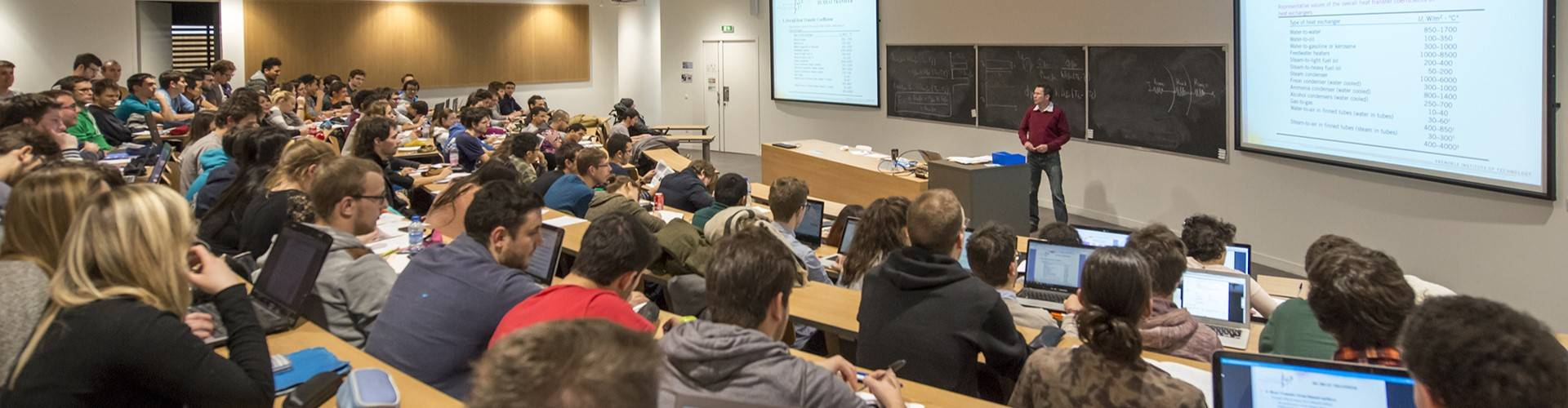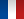# Mathematics for Engineers - 3EUS1MAT

• #### Number of hours

• Lectures 30.0
• Projects -
• Tutorials 30.0
• Internship -
• Laboratory works -

ECTS 5.0

## Goal(s)

To give students mathematical tools in order to represent physical models

Introduction to probabilistic tools and hazardous phenomenon modelings.
Introduction to statistic notions and parameter estimation or statistical hypothesis validation

Responsible(s)

Antoine VEZIER

## Content(s)

Differential equations
Complex variable functions
Fourier transform
Laplace transform
Z transform

Elementary probabilities: problem modeling by creating an universe and a set of events, counting, conditional probabilities.
Random, discrete, continuous variables.
Discrete and continuous random vectors.
Gaussian vectors
Central Limit Theorem

Prerequisites

Knowledge about the complex body, cartesian or polar coordinate calculation, geometrical representation
Classical analysis tools: integer series, integration

Test

One written test of 3h and ongoing assessment during the tutorial sessions

EN 30% + ER 70%

Calendar

The course exists in the following branches:

see the course schedule for 2023-2024

Course language(s):